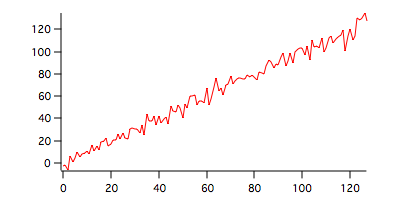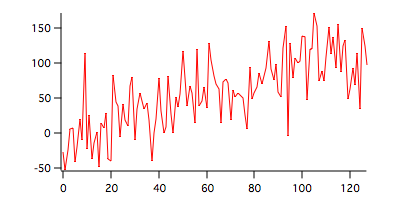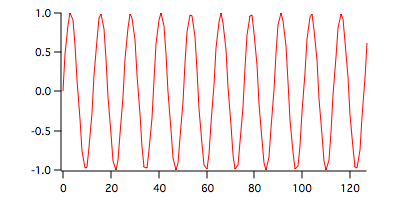The Kendall Tau test computes a correlation coefficient (tau) similar to Spearman's correlation coefficient by ranking all possible pairs of entries. A tau value of 1 implies that the data is ordered perfectly according to rank while a tau value of -1 implies that the data is reverse ordered according to rank. In the examples below we generate various sets of data and compute Kendall's tau.

### 1. Increasing function with low level noise.

To generate the data execute the following command:

`Make wave1=x+gnoise(15)`To compute Kendall's Tau for the data shown above execute the following command:

`StatsKendallTauTest/T=1 wave1`

The results are shown in the table:

 N 128 tau 0.7618 svar 0.00356 zscr 12.753 pValue 2.968e-37

Positive Tau as expected for a generally increasing function.

### 2. Increasing function with high level noise.

To generate the data execute the following command:

`wave1=x+gnoise(35)````StatsKendallTauTest/T=1 wave1

The results are shown in the table:
```
 N 128 tau 0.5366 svar 0.00356 zscr 8.984 pValue 2.598e-19

Positive Tau but smaller than the example above because the level of the noise high relative to the range of x.

### 3. Periodic function

`wave1=sin(x/2)``statsKendallTauTest/T=1 wave1`

The results are shown in the table:

 N 128 au -0.0393701 svar 0.00356791 zscr -0.659112 pValue 0.509824

In this case Tau is close to zero becacuse the function is periodic so the ordering of ranks results in negligible Tau value with a sign that depends on the phase of the periodic signal.ForumSupportGallery